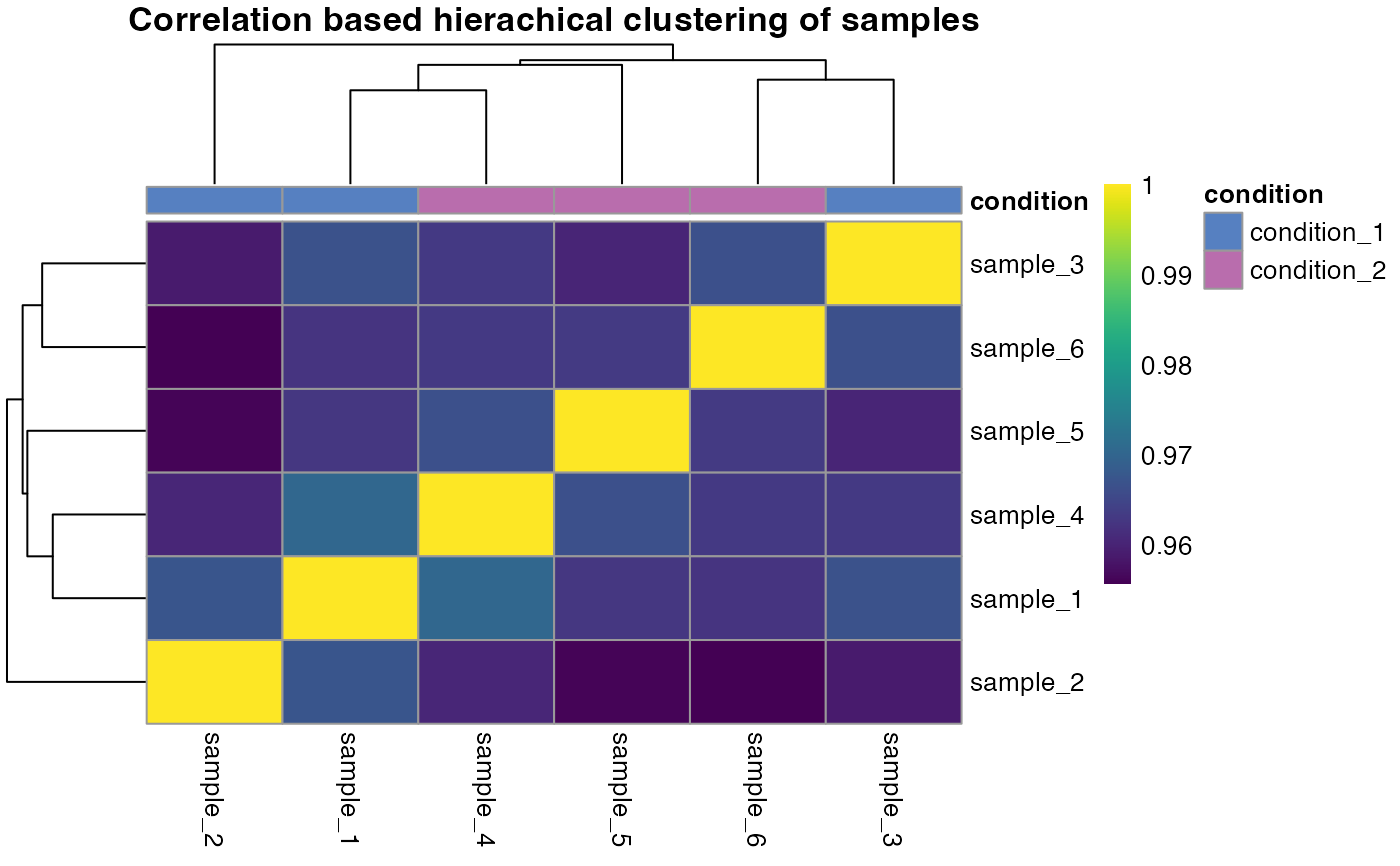A correlation heatmap is created that uses hirachical clustering to determine sample similarity.

qc_sample_correlation(
data,
sample,
grouping,
intensity_log2,
condition,
digestion = NULL,
run_order = NULL,
method = "spearman",
interactive = FALSE
)

## Arguments

data a data frame that contains at least the input variables. a character column in the data data frame that contains the sample names. a character column in the data data frame that contains precursor or peptide identifiers. a numeric column in the data data frame that contains log2 intensity values. a character or numeric column in the data data frame that contains the conditions. optional, a character column in the data data frame that contains information about the digestion method used. e.g. "LiP" or "tryptic control". optional, a character or numeric column in the data data frame that contains the order in which samples were measured. Useful to investigate batch effects due to run order. a character value that specifies the method to be used for correlation. "spearman" is the default but can be changed to "pearson" or "kendall". a logical value that specifies whether the plot should be interactive. Determines if an interactive or static heatmap should be created using heatmaply or pheatmap, respectively.

## Value

A correlation heatmap that compares each sample. The dendrogram is sorted by optimal leaf ordering.

## Examples

set.seed(123) # Makes example reproducible

# Create example data
data <- create_synthetic_data(
n_proteins = 100,
frac_change = 0.05,
n_replicates = 3,
n_conditions = 2,
method = "effect_random"
)

# Create sample correlation heatmap
qc_sample_correlation(
data = data,
sample = sample,
grouping = peptide,
intensity_log2 = peptide_intensity_missing,
condition = condition
)